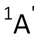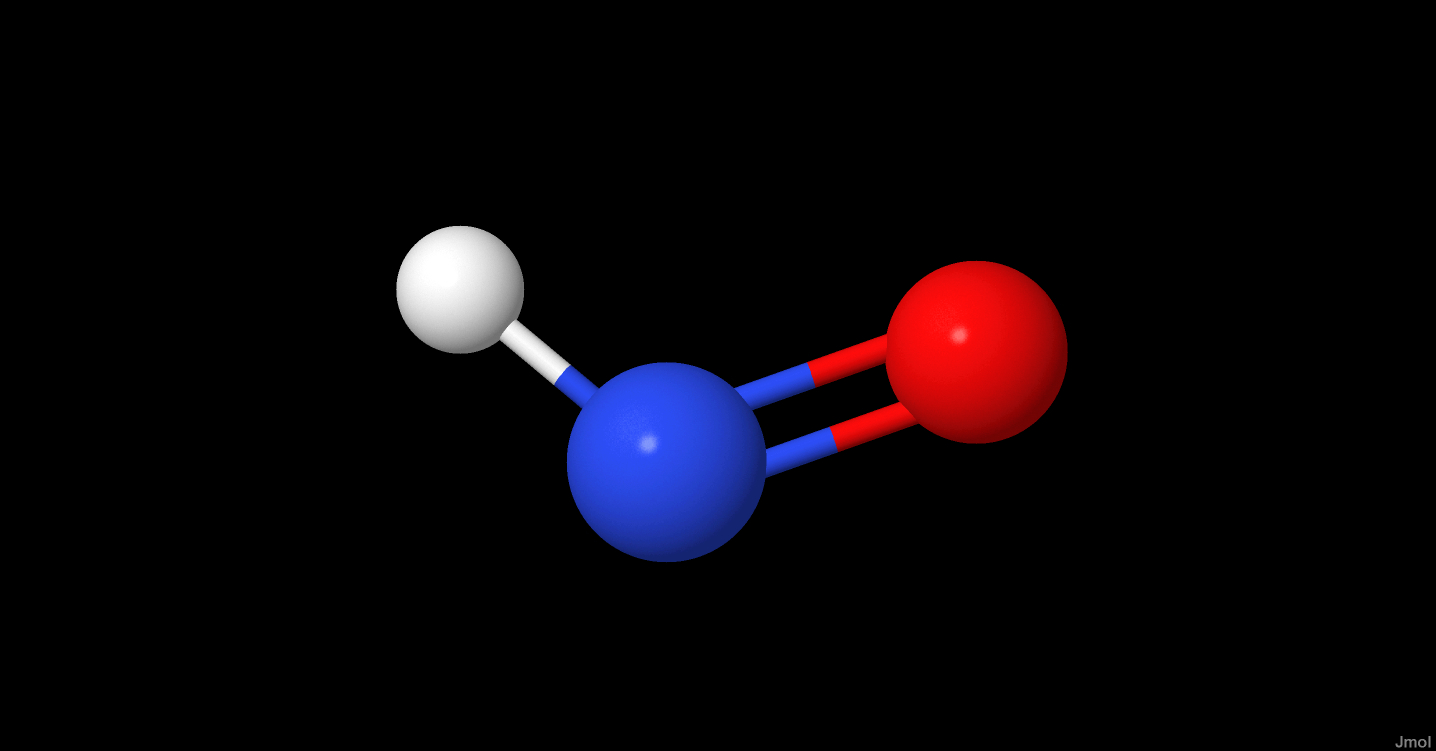# HNO

Click on the   icon info to get details.

Species data
 Common Formula HNO Stoichiometric Formula HNO Name Nitrosyl hydride Mass 31.00581 a.m.u Charge 0 CAS 14332-28-6 Inchi InChI=1S/HNO/c1-2/h1H InchiKey ODUCDPQEXGNKDN-UHFFFAOYSA-N State Ground StateISM Abundance
log10 Abundance Reference Source Name Source Type Link
Polarizability
 Definition: total Value (Å3): 1.846 Method: Calculations Origin: Database : NIST COMPUTATIONAL CHEMISTRY COMPARISON AND BENCHMARK DATABASE Reference:
Dipole moment
 Value (D): 1.67 Method: Measurements Origin: Database : NIST COMPUTATIONAL CHEMISTRY COMPARISON AND BENCHMARK DATABASE Reference:
Enthalpy of formation
 T (K): 0 Value (kJ.mol-1) : 109.809 ±0.125 Method: Reviews and Evaluations Origin: Other database Reference: T (K): 298 Value (kJ.mol-1) : 106.842 ±0.125 Method: Reviews and Evaluations Origin: Other database Reference: T (K): 298 Value (kJ.mol-1) : 107.05 ±0.25 Method: Measurements Origin: Other database Reference: T (K): 0 Value (kJ.mol-1) : 110.02 ±0.25 Method: Measurements Origin: Other database Reference:
Desorption energy
 Emean (K): 3000 ±900 E min (K): 0 E max (K): 0 Pre-exponential factor (s-1): 0.00E+0 Method: Calculations Origin: Bibliography Reference: Wakelam, V. et al. ;2017;ArXiv e-prints;, Type of surface: H2O Description: To estimate the unknown binding energies (for most of the radicals for example), we have developed a model founded on the stabilization energy of the complex between the various species and one water molecule. Then, we assume that the binding energy of the species with ASW is proportional to the energy of interaction between this species and one water molecule. To determine the proportionality coefficients, we fit the dependency of the experimental binding energies versus the calculated energies of the complexes for 16 stable molecules. Uncertainties in ED is estimated to be 30%. The preexponential factor is to be computed using the Hasegawa et al. (1992) approximation. Evaluation: Emean (K): 2050 E min (K): 0 E max (K): 0 Pre-exponential factor (s-1): 0.00E+0 Method: Estimation Origin: Other database Reference: Type of surface: H2O Description: This binding energy was listed in the original OSU gas-grain code from Eric Herbst group in 2006. Energy of H+N+O The pre-exponential factor is not given. It can be computed using the formula given in Hasegawa et al. (1992). Evaluation:
Diffusion energy
No data